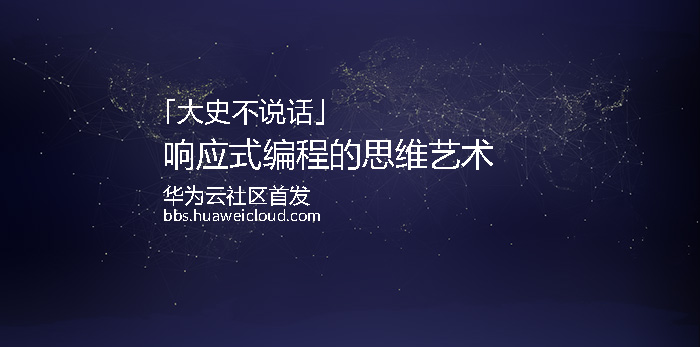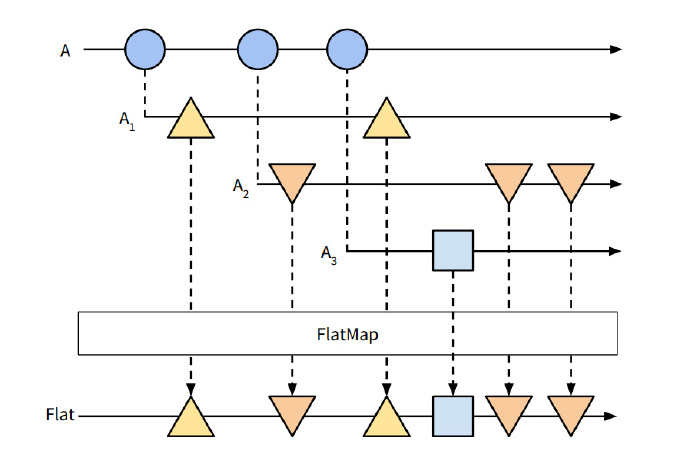## 一. 划重点

• map-映射
• filter-过滤
• reduce-有限列聚合
• scan-无限列聚合
• flatMap-拉平操作（重点）
• catch-捕获错误
• retry-序列重试
• from-生成可观测序列
• range-生成有限的可观测序列
• interval-每隔指定时间发出一次顺序整数
• distinct-去除出现过的重复值

## 二. flatMap功能解析

var quakes = Rx.Observable.create(function(observer) {
//模拟得到的响应流
var response = {
features:[{
earth:1
},{
earth:2
}],
test:1
}
/*  最初的手动遍历代码
var quakes = response.features;
quakes.forEach(function(quake) {
observer.onNext(quake);
});*/

observer.onNext(response);
})
//为了能将features数组中的元素逐个发送给订阅者，需要构建新的流
.map(dataset){
return Rx.Observable.from(dataset.features)
}


quakes.subscribe(function(data){
data.subscribe(function(quake){
console.log(quake);
})
})；var quakes = Rx.Observable.create(function(observer) {
var response = {
features:[{
earth:1
},{
earth:2
}],
test:1
}
observer.onNext(response);
}).flatMap((data)=>{
return Rx.Observable.from(data.features);
});

quakes.subscribe(function(quake) {
console.log(quake)
});


## 三. flatMap的推演

### 3.1 函数式编程基础知识回顾

/*map运算符的作用
*对所有容器类而言，它相当于打开容器，进行操作，然后把容器再盖上。
*Container在这里只是一个抽象定义，为了看清楚它对于容器中包含的值意味着什么。
*你会发现它其实就是Observable的抽象原型。
*/
Container.prototype.map = function(f){
return Container.of(f(this.__value))
}

//基本的科里化函数
var curry = function(fn){
args = [].slice.call(arguments, 1);
return function(){
[].push.apply(args, arguments);
return fn.apply(this, args);
}
}

//map pointfree风格的map运算符
var map = curry(function(f, any_functor_at_all) {
return any_functor_at_all.map(f);
});

/*compose函数组合方法
*运行后返回一个新函数，这个函数接受一个参数。
*函数科里化的基本应用，也是函数式编程中运算管道构建的基本方法。
*/
var compose = function (f, g) {
return function (x) {
return f(g(x));
}
};
/*IO容器
*一个简单的Container实现，用来做流程管理
*这里需要注意，IO实现的作用是函数的缓存，且总是返回新的IO实例
*可以看做一个简化的Promise,重点是直观感受一下它作为函数的
*容器是如何被使用的，对于理解Observable有很大帮助
*/
var IO = function(f) {
this.__value = f;
}

IO.of = function(x) {
return new IO(function() {
return x;
});
}

IO.prototype.map = function(f) {
return new IO(compose(f, this.__value));
}


### 3.2 从一个容器的例子开始

var fs = require('fs');

//读取文件

//转换字符
var transContent = (content)=>IO.of(()=>content.replace('a','b'));

//写入字符串
var writeFile = (content)=>IO.of(()=>fs.writeFileSync('dest.txt',content));


/*
*map(transContent)是一个高阶函数，它的返回函数就可以接收一个容器实例，
*并对容器中的内容执行map操作。
*/


io{
__value:io{
__value:someComposedFnExpression
}
}


var task = compose(map(map(writeFile)),map(transContent),readFile);
//io{io{io{__value:someComposedFnExpression}}}


👉 提示一

👉提示二:

//链式调用风格
IO.prototype.join =  function(){
return this.isNothing() ? IO.of(null):this.__value;
}
//pointfree风格运算符
var join = (m)=>m.join();


var task = compose(join,map(writeFile),join,map(transContent),readFile);


var chain = curry(function(f,m){
return m.map(f).join();
})


var task = compose(chain(writeFile),chain(transContent),readFile);


### 3.4 对比总结

//原有形式

//map-join形式

//chain形式(flatMap)

flatMap所解决问题，是在函数式编程引入了Functor的概念将逻辑函数包裹在容器中后才产生的，那么这种容器概念的引入对函数式编程到底有什么意义，笔者尚未搞清楚，相关内容留作以后补充。